Courses

# NCERT Solutions(Part- 4)- Factorisation Class 8 Notes | EduRev

## Class 8 Mathematics by Full Circle

Created by: Full Circle

## Class 8 : NCERT Solutions(Part- 4)- Factorisation Class 8 Notes | EduRev

The document NCERT Solutions(Part- 4)- Factorisation Class 8 Notes | EduRev is a part of the Class 8 Course Class 8 Mathematics by Full Circle.
All you need of Class 8 at this link: Class 8

EXERCISE 14.4
Question: Find and correct the errors in the following mathematical statements.
1. 4(x – 5) = 4x – 5
2. x(3x + 2) = 3x2 + 2
3. 2x + 3y = 5xy
4. x + 2x + 3x = 5x
5. 5y + 2y + y – 7y = 0
6. 3x + 2x = 5x2
7. (2x)+ 4(2x) + 7 = 2x+ 8x + 7
8. (2x)2 + 5x = 4x + 5x = 9x2
9. (3x + 2)2 = 3x2 + 6x + 4
10. Substituting x = – 3 in
(a) x2 + 5x + 4 gives (–3)2 + 5(–3) + 4 = 9 + 2 + 4 = 15
(b) x2 – 5x + 4 gives (–3)2 – 5(–3) + 4 = 9 – 15 + 4 = – 2
(c) x2 + 5x + 4 gives (–3)2 + 5(–3) = – 9 – 15 = – 24
11. (y – 3)2 = y– 9
12. (z + 5)2 = z2 + 25
13. (2a + 3b)(a – b) = 2a2 – 3b2
14. (a + 4)(a + 2) = a2 + 8
15. (a – 4)(a – 2) = a2 – 8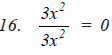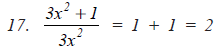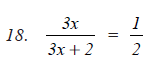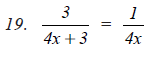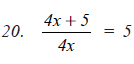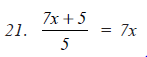Solution:
1. 4(x – 5) = 4x – 5
The given statement is incorrect.
The correct statement is:
4(x – 5) = 4x – 20                           (∵ 4 * 5 = 20)

2. x(3x + 2) = 3x2 + 2
It is an incorrect statement.
The correct statement is:
x(3x + 2) = 3x2 + 2x

3. 2x + 3y = 5xy
It is an incorrect statement.
The correct statement is:
2x + 3y = 2x + 3y

4. x + 2x + 3x = 5x
∵ 1 + 2 + 3 = 5 is an incorrect statement.
∴ The correct statement is:
x + 2x + 3x = 6x

5. 5y + 2y + y – 7y = 0
It is an incorrect statement.
∵ 5y + 2y + y = 8y and 8y – 7y = y
∴ The correct statement is
5y + 2y + y – 7y = y

6. 3x + 2x = 5x2
It is an incorrect statement.
The correct statement is:
3x + 2x = 5x

7. (2x)2 + 4(2x) + 7= 2x2 + 8x + 7
∵ (2x)2 = 4x2
∴ The given statement is incorrect.
The correct statement is:
(2x)2 + 4(2x) + 7 = 4x2 + 8x + 7

8. (2x)2 + 5x = 4x + 5x = 9x, is an incorrect statement.
∵ (2x)2 = 4x2
∴ The correct statement is:
(2x)2 + 5x = 4x2 + 5x

9. (3x + 2)2= 3x2 + 6x + 4
The given statement is incorrect.
∵ (3x + 2)2 = (3x)2 + 2(3x)(2) + (2)2
= 9x2 + 6x + 4
∴ The correct statement is:
(3x + 2)2 = 9x2 + 6x + 4

10. (a) Incorrect statement.
∵ x2 + 5x + 4 = (–3)2 + 5(–3) + 4
= 9 – 15 + 4
= (9 + 4) – 15
= 13 – 15 = –2
Thus, the correct statement is:
x2 + 5x + 4 = (–3)2 + 5(–3) + 4
= 9 – 15 + 4 = –2

(b) We have
x2 – 5x + 4 = (–3)2 – 5(–3) + 4
= 9 + 15 + 4
= 28
∴ The correct statement is
x2 – 5x + 4 at x = –3 is
(–3)2 – 5(–3) + 4 = 9 + 15 + 4 = 28

(c) ∵   x2 + 5x at x = – 3 is
(–3)2 + 5(–3) = 9 – 15 = –6
∴ The correct statement is
x2 + 5x at x = –3 is
(–3)2 + 5(–3) = 9 – 15 = –6

11. (y – 3)2 = y2 – 9
The given statement is incorrect.
∵             (y – 3)2 = y2 – 2(y)(3) + (3)2 = y2 – 6y + 9
The correct statement is
(y – 3)2 = y2 – 6y + 9

12. (z + 5)2 = z2 + 25
The given statement is incorrect.
∵     (z + 5)= z2 + 2(z)(5) + (5)2
= z2 + 10z + 25
∴ The correct statement is
(z + 5)2 = z2 + 10z + 25

13.  (2a + 3b)(a – b) = 2a2 – 3b2
∵      (2a + 3b) (a – b) = a(2a + 3b) – b (2a – 3b)
= 2a2 + 3ab – 2ab – 3b2
= 2a2 + ab – 3b2
∴ The correct statement is
(2a + 3b)(a – b) = 2a2 + ab + 3b2

14.  (a + 4)(a + 2) = a2 + 8
Since (a + 4) (a + 2) = a (a + 4) + 2 (a + 4)
= a2 + 4a + 2a + 8
= a2 + 6a + 8

15.  (b – 4)(a – 2) = a2– 8
Since (a – 4)(a – 2) = a(a – 2) – 4(a – 2)
= a2 – 2a – 4a + 8
= a2 – 6a + 8
∴ The correct statement is
(a – 4)(a – 2) = a2 – 6a + 8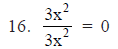It is an incorrect statement.
∵ The correct statement is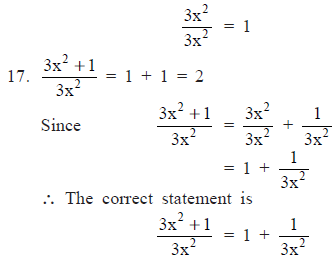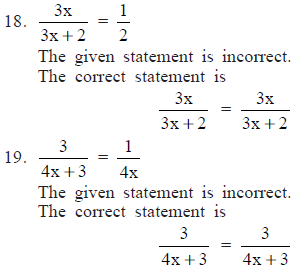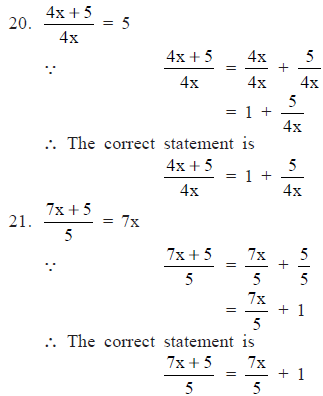93 docs|16 tests

,

,

,

,

,

,

,

,

,

,

,

,

,

,

,

,

,

,

,

,

,

;